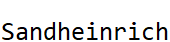#Function Repository Resource:

# Bob

Make anything bob up and down

Contributed by: Bob Sandheinrich
 ResourceFunction["Bob"][expr] displays a bobbing version of expr. ResourceFunction["Bob"][expr,rate] bobs at the specified rate. ResourceFunction["Bob"][expr,rate,size] bobs with the specified size.

## Details and Options

The default rate and size are each 1.
ResourceFunction["Bob"] accepts the option UpdateInterval with a default value of 0.05.
The result of ResourceFunction["Bob"] is a ResourceFunction["Bob"] object.

## Examples

### Basic Examples

Bob something:

 In:=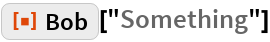Out=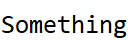Bob it fast and slow:

 In:=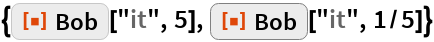Out=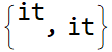Bob it big and small:

 In:=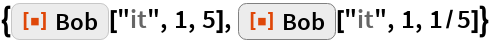Out=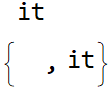### Scope

Bob the callouts on a plot:

 In:=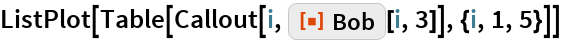Out=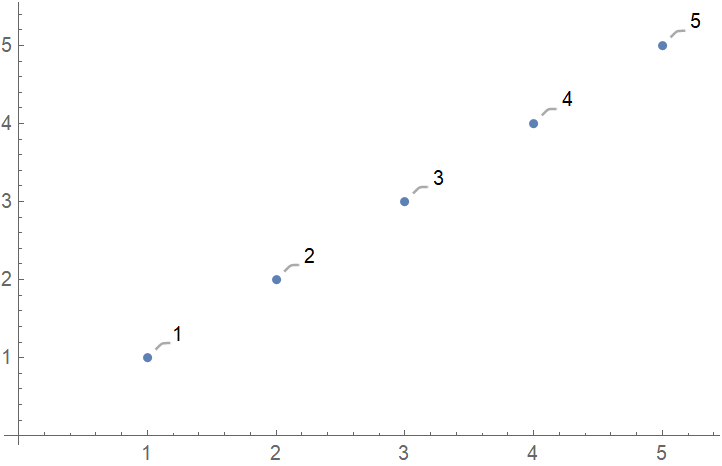Bob one image next to another:

 In:=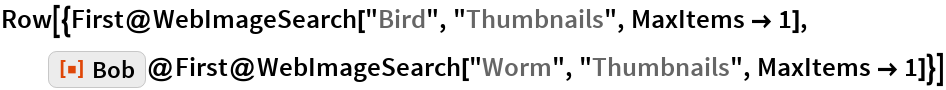Out=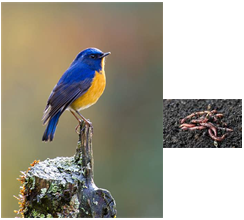This bird can really move its voice up and down:

 In:=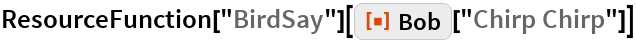Out=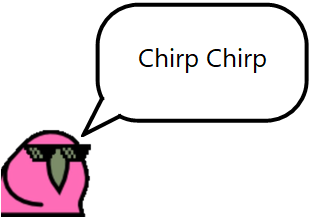### Options

Specify an UpdateInterval:

 In:=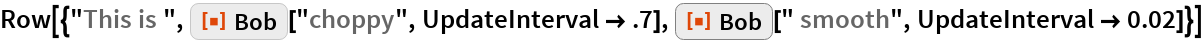Out=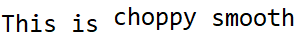### Properties and Relations

The result is a wrapped in Bob:

 In:=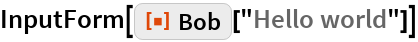Out=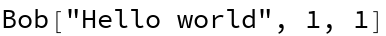In:=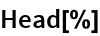Out=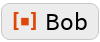As of version 12, there are only two top-level Wolfram Language symbols which are non-trivial palindromes:

 In:=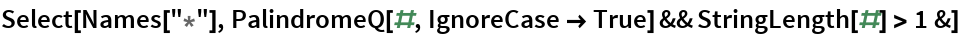Out=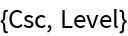Bob joins their ranks from within the Function Repository:

 In:=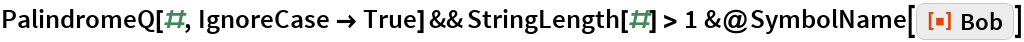Out=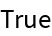### Neat Examples

I am Bob Sandheinrich, and so can you!

 In:=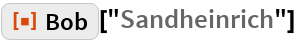Out=